# -3x? – x +11. Given g(x)=:Determineshow algebraically that g'(x) =2V#(x° +x+1}°the x-value(s) of any horizontal tangents. *Hint: The domain of g(x) is important!x² +x+1

Question
60 views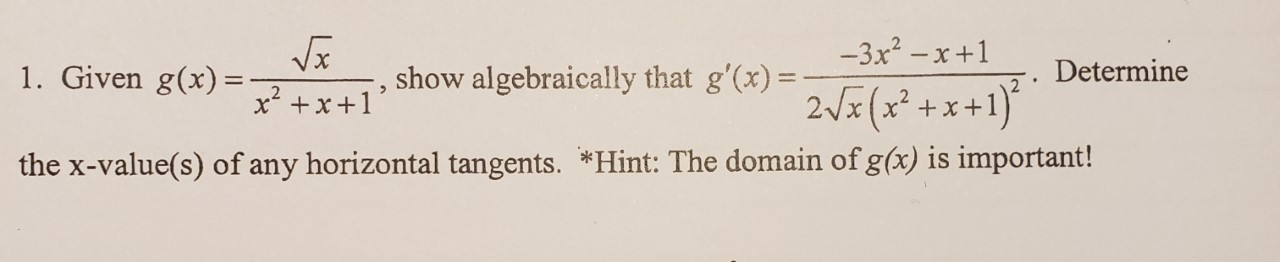help_outlineImage Transcriptionclose-3x? – x +1 1. Given g(x)=: Determine show algebraically that g'(x) = 2V#(x° +x+1}° the x-value(s) of any horizontal tangents. *Hint: The domain of g(x) is important! x² +x+1 fullscreen
check_circle

Step 1

Given the function,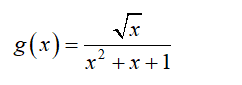To calculate the derivative of function g(x), apply the quotient rule formula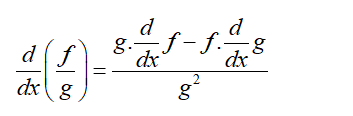Differentiate the function with respect to x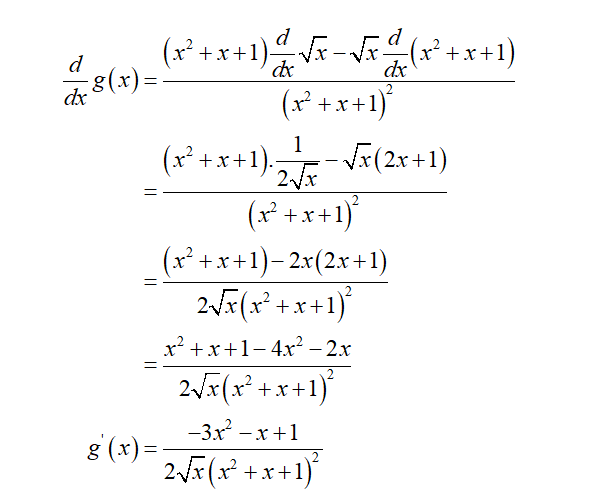Step 2

The x values of any horizontal tangent,

Substitute the value of derivatives of function is equal to zero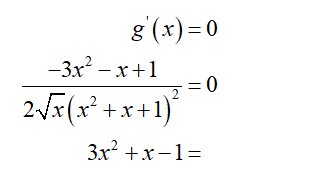Solve the quadratic equation for value x

Compare the equation with the standard equation,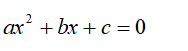Substitute the value of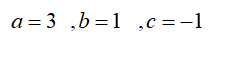...

### Want to see the full answer?

See Solution

#### Want to see this answer and more?

Solutions are written by subject experts who are available 24/7. Questions are typically answered within 1 hour.*

See Solution
*Response times may vary by subject and question.
Tagged in